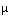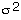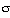Footnote

In statistics, the collection of n examples is called a sample of size n. Strictly speaking, m is the sample mean and v is the sample variance. The exact mean is the sample mean for an infinitely large collection, and similarly for the variance. We will be a little careless here and refer to the sample mean as the mean.

Also, it is more conventional to denote the mean byand the variance by, whereis called the standard deviation. We use m, v ans s merely because Roman symbols are easier to enter in HTML.Go back